GeeksforGeeks App
Open AppBrowser
Continue

# How To Make Violin Plots with ggplot2 in R?

Violin plots help us to visualize numerical variables from one or more categories. They are similar to box plots in the way they show a numerical distribution using five summary-level statistics. But violin plots also have the density information of the numerical variables. It allows visualizing the distribution of several categories by displaying their densities.

In this article, we will discuss how to plot a violin plot with the help of the ggplot2 library in R Programming Language. To plot a violin plot using the ggplot2 package we use the geom_violin() function.

Syntax: ggplot( dataframe, aes( x, y, fill, color)) + geom_violin()

Parameters:

• dataframe: determines the dataset used in the plot.
• fill: determines the color of background of interior of the plot.
• color: determines the color of boundary of plot.

## Creating basic Violin Plots

Here, is a basic violin plot made using the geom_violin() function. We have used the diamonds data frame in this plot which is provided by the R language natively.

## R

 `# load library ggplot2``library``(ggplot2)` `# Basic violin plot``# diamonds dataframe has been used here``# diamonds dataframe is provided by R language natively.``ggplot``(diamonds, ``aes``(x=cut, y=price)) +` `# geom_violin() function is used to plow violin plot``  ``geom_violin``()`

Output: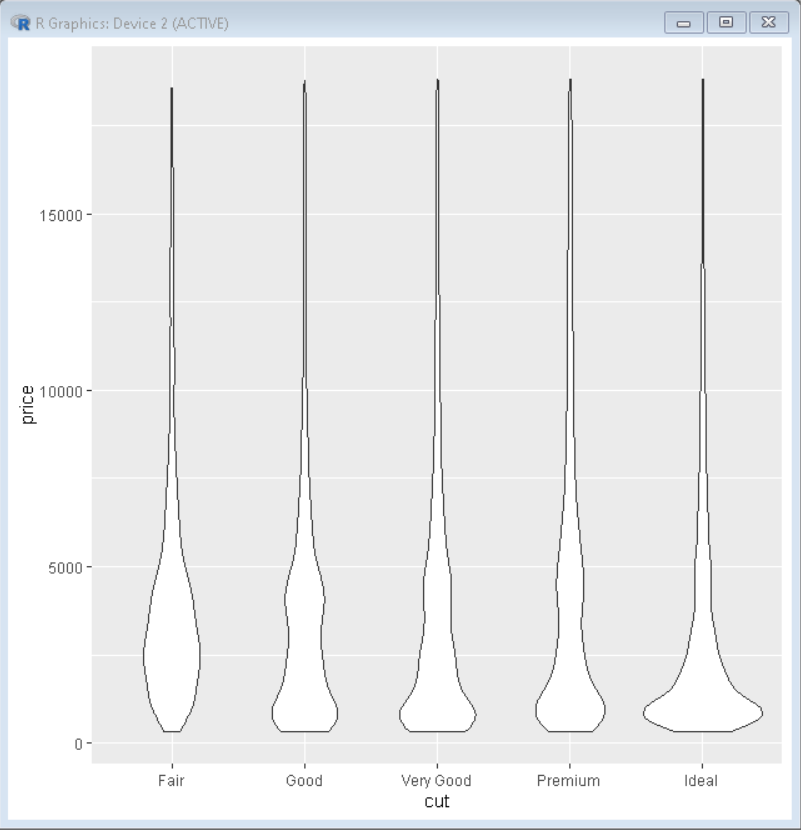## Color Customization

We can change the color of the violin plot using the color parameter of aes() function of ggplot2. This changes the color of the boundary of the violin plot according to the category of data. Here, plots are colored according to the category of their cut by putting cut as parameter color.

## R

 `# load library ggplot2``library``(ggplot2)` `# Basic violin plot``# diamonds dataframe has been used here``# diamonds dataframe is provided by R language natively``# color parameter is used to color the boundary of``# plot according to category``ggplot``(diamonds, ``aes``(x=cut, y=price, color=cut)) +` `# geom_violin() function is used to plow violin plot``  ``geom_violin``()`

Output: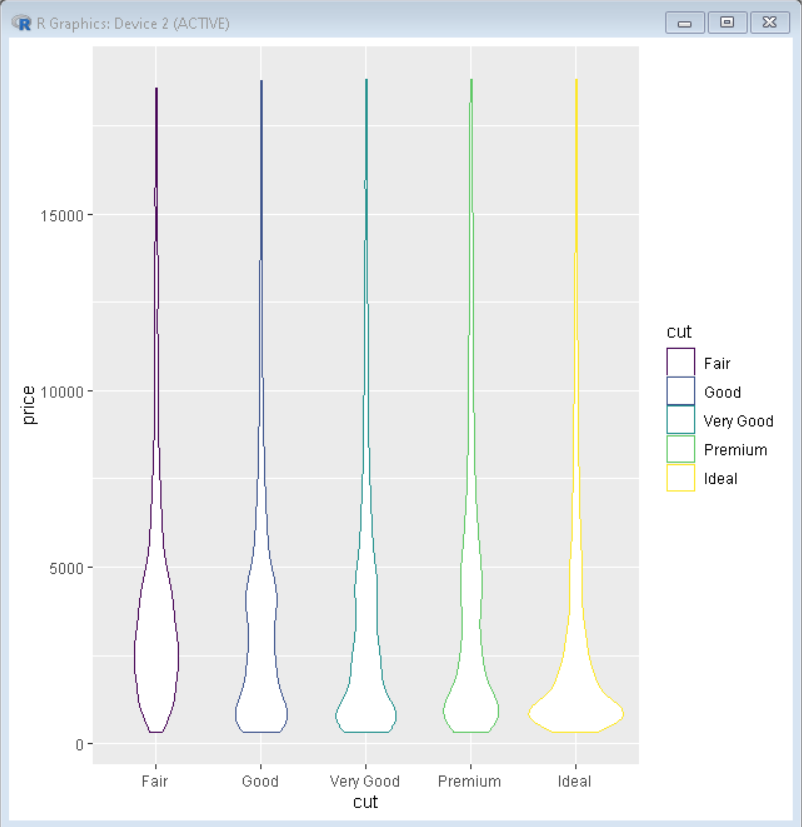We can change the background color of the violin plot using the fill parameter of aes() function of ggplot2. This changes the color of the background of the interior of the violin plot according to the category of data.

Here, plots are colored according to the category of their cut by putting cut as parameter fill.

## R

 `# load library ggplot2``library``(ggplot2)` `# Basic violin plot``# diamonds dataframe has been used here``# diamonds dataframe is provided by R language natively``# fill parameter is used to color the background of``#plot according to category``ggplot``(diamonds, ``aes``(x=cut, y=price, fill=cut)) +` `# geom_violin() function is used to plow violin plot``  ``geom_violin``()`

Output: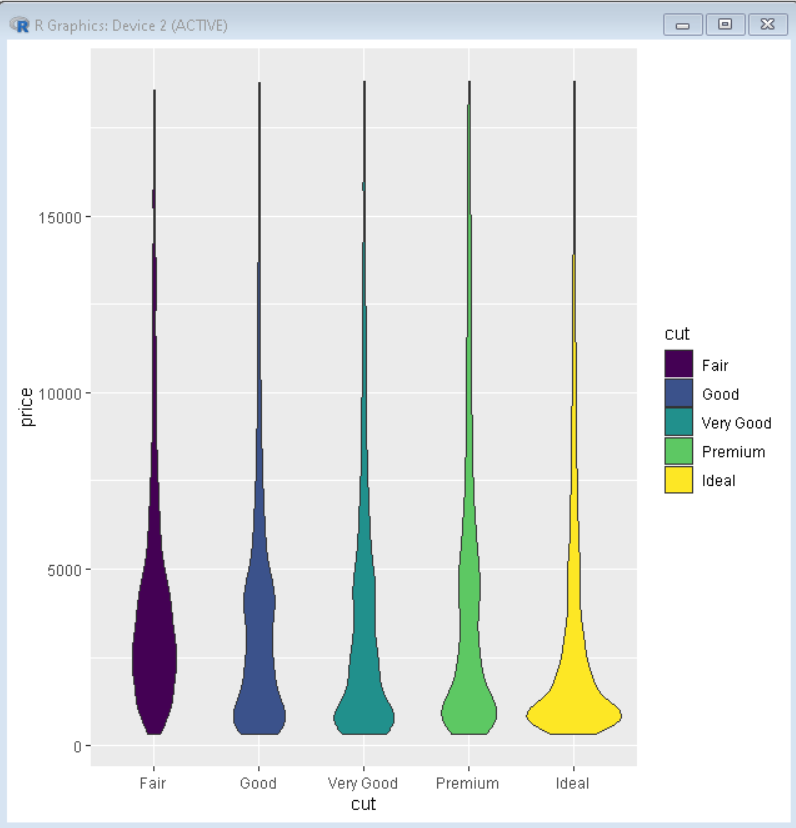## Horizontal Violin Plot

To convert a normal violin plot to a horizontal violin plot we add coord_flip() function to the ggplot() function. This flips the coordinate axis of the plot and converts any ggplot2 plot into a horizontal plot.

Syntax: plot+ coord_flip()

Here, is a horizontal violin plot made using coord_flip() function.

## R

 `# load library ggplot2``library``(ggplot2)` `# Horizontal violin plot``# diamonds dataframe has been used here``# diamonds dataframe is provided by R language natively.``ggplot``(diamonds, ``aes``(x=cut, y=price)) +` `# geom_violin() function is used to plow violin plot``geom_violin``()+` `# coord_flip() function is used to make horizontal``# violin plot``coord_flip``()`

Output: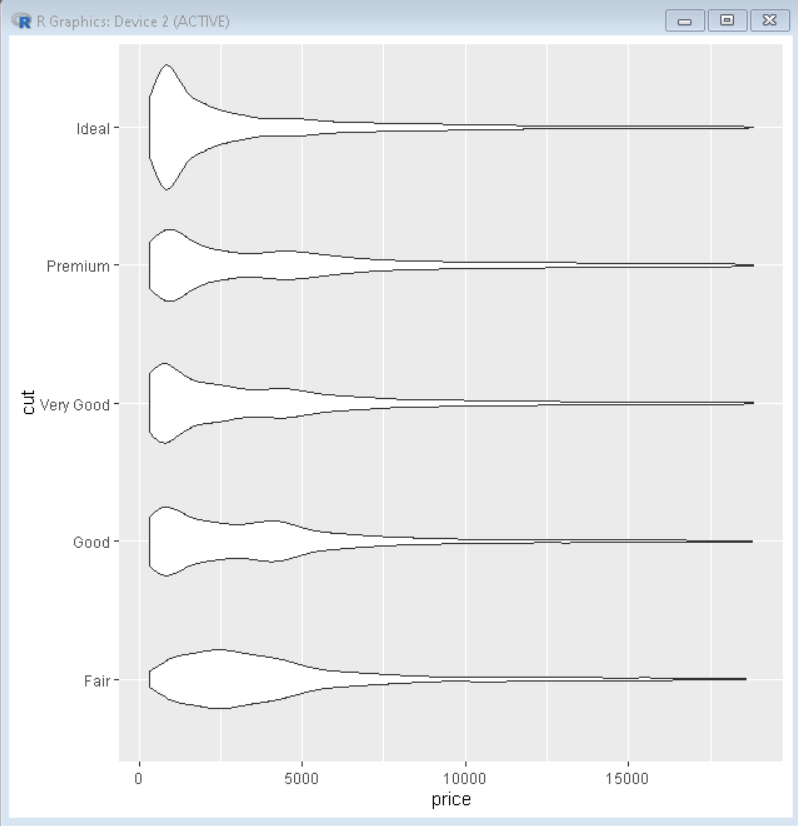## Mean marker customization

In ggplot2, we use the stat_summary() function to compute new summary statistics and add it to the plot. We use stat_summary() function with ggplot() function.

Syntax:

plot+ stat_summary(fun.y, geom, size, color)

Here,

• fun.y: determines the function according to which marker has to be placed i.e. mean, median, etc.
• geom: determines the shape of marker
• size: determines size of marker
• color: determines the color of marker

Example:

In this example, we will compute the mean value of the y-axis variable using fun.y argument in the stat_summary() function.

## R

 `# load library ggplot2``library``(ggplot2)` `# Basic violin plot``# diamonds dataframe has been used here``# diamonds dataframe is provided by R``# language natively.``ggplot``(diamonds, ``aes``(x=cut, y=price)) +` `# geom_violin() function is used to plow violin plot``  ``geom_violin``()+` `# Stat_summary() function adds mean marker on plot``stat_summary``(fun.y=``"mean"``, geom=``"point"``, size=2, color=``"red"``)`

Output: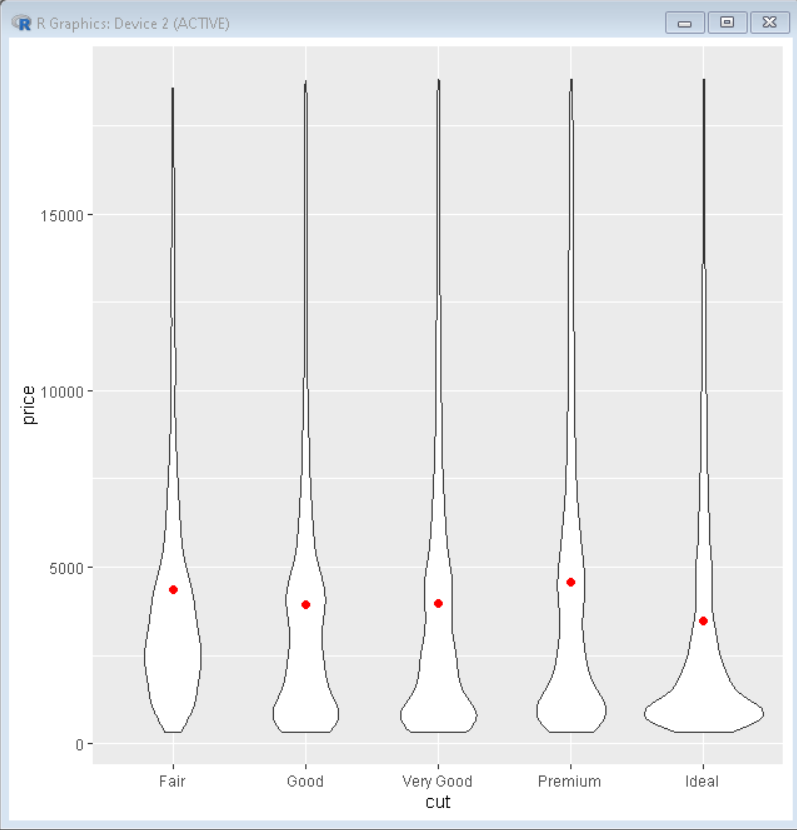Here, the point in the center of the violin shows the variation of the mean of the y-axis for each category of data on the x-axis.

My Personal Notes arrow_drop_up